# Getting Started with NNS: Correlation and Dependence

library(NNS)
library(data.table)
require(knitr)
require(rgl)
require(meboot)

# Correlation and Dependence

The limitations of linear correlation are well known. Often one uses correlation, when dependence is the intended measure for defining the relationship between variables. NNS dependence NNS.dep is a signal:noise measure robust to nonlinear signals.

Below are some examples comparing NNS correlation NNS.cor and NNS.dep with the standard Pearson’s correlation coefficient cor.

## Linear Equivalence

Note the fact that all observations occupy the co-partial moment quadrants.

x = seq(0, 3, .01) ; y = 2 * x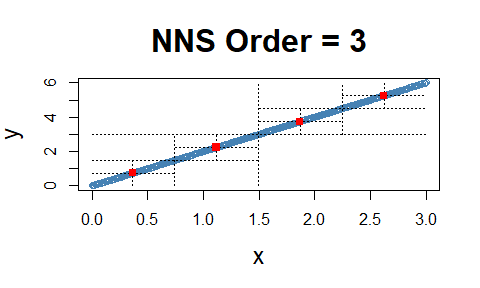cor(x, y)
##  1
NNS.dep(x, y)
## $Correlation ##  1 ## ##$Dependence
##  1

## Nonlinear Relationship

Note the fact that all observations occupy the co-partial moment quadrants.

x = seq(0, 3, .01) ; y = x ^ 10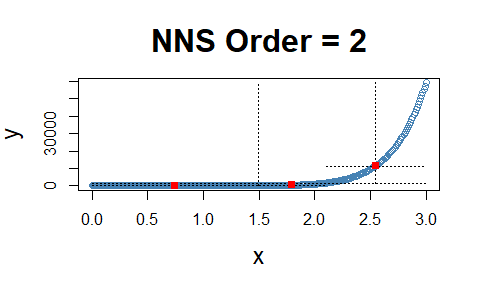cor(x, y)
##  0.6610183
NNS.dep(x, y)
## $Correlation ##  0.9880326 ## ##$Dependence
##  0.9998937

## Cyclic Relationship

Even the difficult inflection points, which span both the co- and divergent partial moment quadrants, are properly compensated for in NNS.dep.

x = seq(0, 12*pi, pi/100) ; y = sin(x)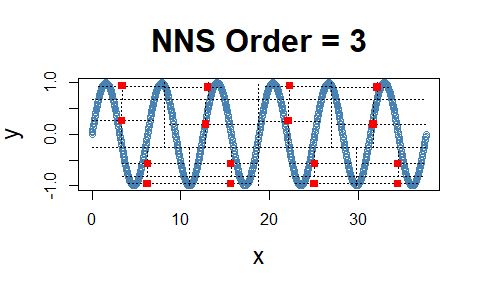cor(x, y)
##  -0.1297766
NNS.dep(x, y)
## $Correlation ##  -0.002982095 ## ##$Dependence
##  0.9999998

## Dependence

Note the fact that all observations occupy only co- or divergent partial moment quadrants for a given subquadrant.

set.seed(123)
df <- data.frame(x = runif(10000, -1, 1), y = runif(10000, -1, 1))
df <- subset(df, (x ^ 2 + y ^ 2 <= 1 & x ^ 2 + y ^ 2 >= 0.95))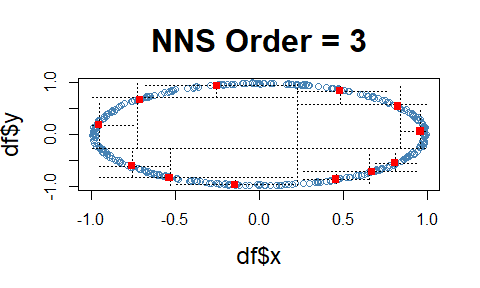NNS.dep(df$x, df$y)
## $Correlation ##  0.02524717 ## ##$Dependence
##  0.9830499

# p-values for NNS.dep()

p-values and confidence intervals can be obtained from sampling random permutations of $$y \rightarrow y_p$$ and running NNS.dep(x,$y_p$) to compare against a null hypothesis of 0 correlation, or independence between $$(x, y)$$.

Simply set NNS.dep(..., p.value = TRUE, print.map = TRUE) to run 100 permutations and plot the results.

## p-values for [NNS.dep]
x <- seq(-5, 5, .1); y <- x^2 + rnorm(length(x))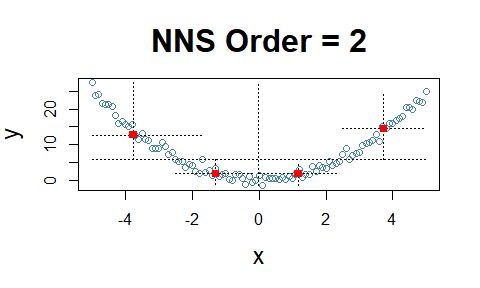NNS.dep(x, y, p.value = TRUE, print.map = TRUE)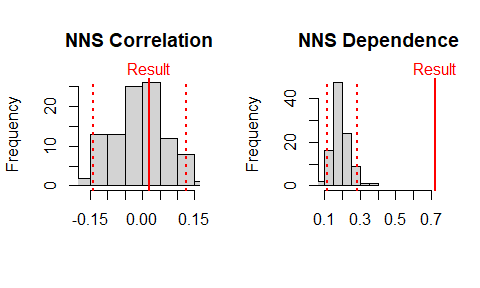## $Correlation ##  0.01943686 ## ##$Correlation p.value
##  0.34
##
## $Correlation 95% CIs ## 2.5% 97.5% ## -0.1391829 0.1246556 ## ##$Dependence
##  0.7206435
##
## $Dependence p.value ##  0 ## ##$Dependence 95% CIs
##      2.5%     97.5%
## 0.1131909 0.2852342

# Multivariate Dependence NNS.copula()

These partial moment insights permit us to extend the analysis to multivariate instances and deliver a dependence measure $$(D)$$ such that $$D \in [0,1]$$. This level of analysis is simply impossible with Pearson or other rank based correlation methods, which are restricted to bivariate cases.

set.seed(123)
x <- rnorm(1000); y <- rnorm(1000); z <- rnorm(1000)
NNS.copula(cbind(x, y, z), plot = TRUE, independence.overlay = TRUE)
##  0.09571785

# Simulating a Multivariate Dependence Structure

Analogous to an empirical copula transformation, we can generate new data from the dependence structure of our original data via the following steps:

• Determine the dependence structure:

This is accomplished using LPM.ratio(1, x, x) for continuous variables, and LPM.ratio(0, x, x) for discrete variables, which are the empirical CDFs of the marginal variables.

• Generate or supply new data:

new data must be of equal dimensions to original data. new data does not have to be of the same distribution or dimension as the original data, nor does each dimension of new data have to share a distribution type.

• Apply dependence structure to new data:

We then utilize LPM.VaR(...) to ascertain new data values corresponding to original data position mappings, and return a matrix of these transformed values with the same dimensions as new.data.

# Add variable x to original data to avoid total independence (example only)
original.data <- cbind(x, y, z, x)

# Determine dependence structure
dep.structure <- apply(original.data, 2, function(x) LPM.ratio(1, x, x))

# Generate new data with different mean and sd (or distribution type or dimensions)
new.data <- sapply(1:ncol(original.data), function(x) rnorm(dim(original.data), mean = 10, sd = 20))

# Apply dependence structure to new data
new.dep.data <- sapply(1:ncol(original.data), function(x) LPM.VaR(dep.structure[,x], 1, new.data[,x]))

## Compare Multivariate Dependence Structures

NNS.copula(original.data)
##  0.4360284
NNS.copula(new.dep.data)
##  0.4390859